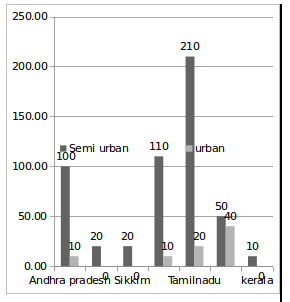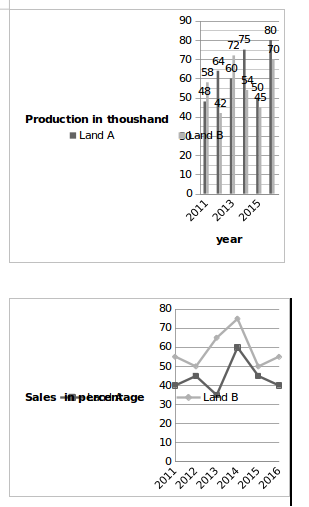## Important Data Interpretation For SBI PO : Set -70

D.1-5) The following line and bar graphs show the number of POS centres in urban, semi-urban and rural areas of different states in the year 2017. Refer to the graphs to answer the questions that follow.1) If the POS centres have increased at a steady rate of 25% in all states of India, then what will be the number of POS centres in Andhra Pradesh in the year 2018?

a) 1050

b) 950

c) 850

d) 750

e) 650

b)

Total POS centres in Andhra pradesh in year 2017 = 100 + 10 + 650 = 760

Hence, the total number of POS centres in Andhra pradesh in year 2018

760(1+25/100)^1= 950

2) If Kerala Government decided to increase POS centres in rural areas at 25% per year and in semi-urban areas at 20% per year, then what will be the total number of POS centres in Kerala in 2018?

a) 80

b) 50

c) 87

d) 82

e) 31

c)

The number of POS centres in rural areas of Kerala in year 2017 = 60

The number of POS centres in rural areas of Kerala in year 2018

=60(1+25/100)^1=75

The number of POS centres in semi-urban areas of Kerala in year 2017 = 10

The number of POS centres in semi-urban areas of Kerala in year 2018

=10(1+20/100)^1=12

Hence, the total number of POS centres in Kerala in year 2018 = 75+12 =87.

3) If in 2017 the average number of POS centres in rural areas of Andhra Pradesh, Telegana, Sikkim, Mizoram, Tamilnadu, Bihar, Kerala and Gujarat is 670, then the number of POS centres in rural areas of Gujarat will be:

a) 790

b) 400

c) 820

d) 500

e) 630

a)

The number of POS centres in rural areas of Gujarat

= (670 × 8) – (650 + 620 + 460 + 1020 + 1750 + 10 + 60)

= 5360 – 4570

= 790.

4) In the year 2019, the Government decided to increase the POS centres according to the population increase in that particular state. If the semi-urban population of Mizoram has increased by 30% from 2017, then what will be the number of POS centres that the Government has to increase in the year 2019 in semi-urban parts of Mizoram?

a) 122

b) 143

c) 150

d) 138

e) 141

b)

Because the population has increased by 30% from 2017 to 2019,

The POS centres have also increased by 30%.

The number of POS centres in semi-urban parts of Mizoram in the year 2019

=110×130/100=143

Increase in the number of POS centres = 143

5) In which of the following states the ratio of rural POS centres to remaining POS centres the second lowest?

a) Bihar

b) Mizoram

d) Telegana

e) Kerala

c)

In Mizoram ratio of rural POS centres is

1020/(110+10)=102/12

For Sikkim =460/20=46/2

For Telegana =620/20=62/2

For Kerala =60/10=6

For Bihar =10/9=1/9

Andhra Pradesh has the second lowest ratio of the rural POS centres with respect to the total POS centres.

D.6-10) Following bar graph shows the production of a grains (In thousands) by two Agriculture land A and B over the years. The line graph shows the percentage sale of these Agriculture products in given years6) what is the difference between average grains produced by land A and land B in the whole period of six years?

a) 2

b) 3

c) 6

d) 5

e) 4

c)

Total number of grains produced by the land A =((48+64+60+75+50+80))/6=377/6=62.83

Total number of grains produced by the land B =((58+42+72+54+45+70))/6=341/6=56.83

Average difference = 62.83 – 56.83 = 6

7) What is the average (approx) sale from land B together in the whole six years?

a) 33533

b) 35333

c) 36533

d) 32333

e) 34533

a)

In 2011 land B Sale is = 58×55/100=31.9

In 2012 land B Sale is = 42×50/100=21

In 2013 land B Sale is = 72×65/100=46.8

In 2014 land B Sale is = 54×75/100=40.5

In 2015 land B Sale is = 45×50/100=22.5

In 2011 land B Sale is = 70×55/100=38.5

Average sale =(31.9+21+46.8+40.5+22.5+38.5)/6= 33533

8) In the following years which year the percentage rise/fall in production of Land B is maximum?

a) 2012

b) 2013

c) 2014

d) 2015

e) 2016

b)

Production in the year 2012 = 42000

Production in the year 2013 = 72000

Required percentage increases =(72-42 )/42 ×100=71.428%

Percentage rise is maximum in the year 2013

9) Sale of grains from Land B in year 2012 is approximately what percentage of sale of grain land A in year 2015?

a) 64%

b) 72%

c) 78%

d) 93%

e) 96%

d)

Sale of grains from Land B in year 2012 = 42×50/100=21000

sale of grain from land A in year 2015 =50×45/100=22500

Required percentage =21000/22500×100=93%

10) Sale of grains from Land A in year 2016 is approximately how much percentage more or less than the sale of grains from Land B in that year?

a) 24%

b) 20%

c) 25%

d) 26%

e) 31%